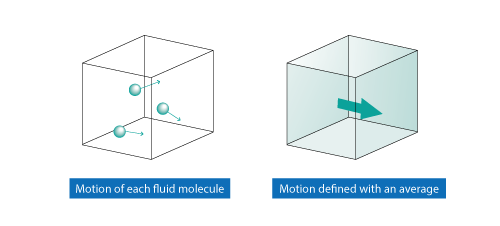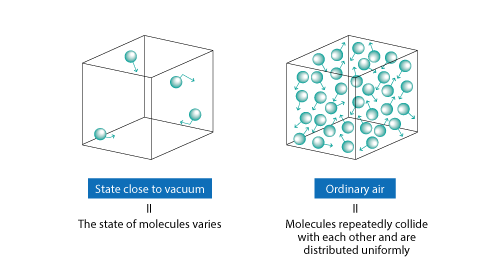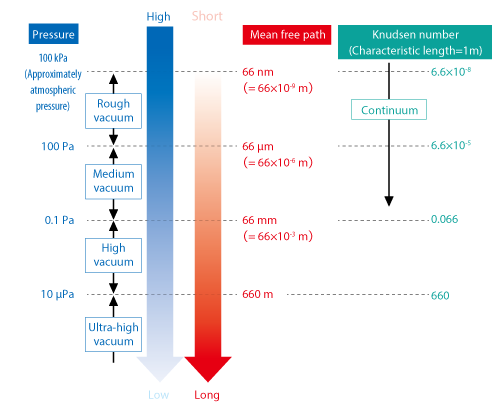# Want to Know More! Basics of Thermo-Fluid Analysis 11: Chapter 3 Flow 3.1 Framework of Fluid Dynamics

#### 3.1 Framework of Fluid Dynamics

Fluid dynamics is an academic discipline of fluid motions.

A substance such as air and water consists of so many molecules and the motion of them cause the flow of a fluid. Their motion vary in each molecule. However, it is difficult to discuss a phenomenon in a microscopic perspective. So, in fluid dynamics, the motion of each molecule is not investigated and a phenomenon is described with an average in a macroscopic perspective.

Figure 3.1 shows an example of a flow. Strictly speaking, velocity of each molecule varies as shown in the figure on the left. In fluid dynamics, a flow is expressed with an average of molecules in a volume as shown in a figure on the right.Figure 3.1 Expression of a flow

A continuum is a substance where the aggregate of molecules is regarded as continuous by using an average of molecules occupying a space. The idea of a continuum is used not only for a fluid but also for a solid. A discipline of the motion of continuums is called continuum mechanics.

Want to know more  Conditions of a continuum
Molecules of a fluid continually move. When the number of molecules in a space is greater than a certain number, molecules repeatedly collide with each other and the distribution of molecules can be regarded as uniform.

However, when the number of molecules is small, the frequency of their collision decreases and molecules are not distributed uniformly. This means that, for air, the level of a vacuum is high. In this case, note that the state of each molecule varies and the approximation as a continuum is not viable.Figure 3.2 State of air and molecules

Knudsen number is an index of whether the approximation of a continuum is viable or not. The number is a dimensionless number defined as the ratio of the molecular mean free path to a characteristic length. Smaller Knudsen number means that molecules collide frequently and are close to being in the state of an assumption of a continuum.
Mean free path is the distance traveled by a moving molecule between successive collisions. This length scale is a length that characterizes a flow (e.g., the radius of a circular tube).

The rough standard value depends on literatures; at any rate, when Knudsen number is smaller than 0.01, we can say that an object is a continuum.

At the end of the section, let us think about the mean free path of air in a vacuum. A vacuum is classified into four pressure regions depending on its level by Japanese Industrial Standards (JIS). When the diameter of a molecule of air is 372 pm (372×10-12 m), and temperature is 20°C, the mean free path in each region is as shown in Figure 3.3.Figure 3.3 Pressure and mean free path

At the boundary between the high vacuum region and the ultra-high vacuum region (10 μPa), the mean free path of air is approximately 660 m. This means that the number of molecules are so small (air is thin) and a molecule can travel 660 m without colliding with another molecule. When the air is filled in a cube box whose one side is 1 m, the air can be regarded as a continuum down to the medium vacuum region. When the pressure is lower, the assumption of a continuum is not viable.